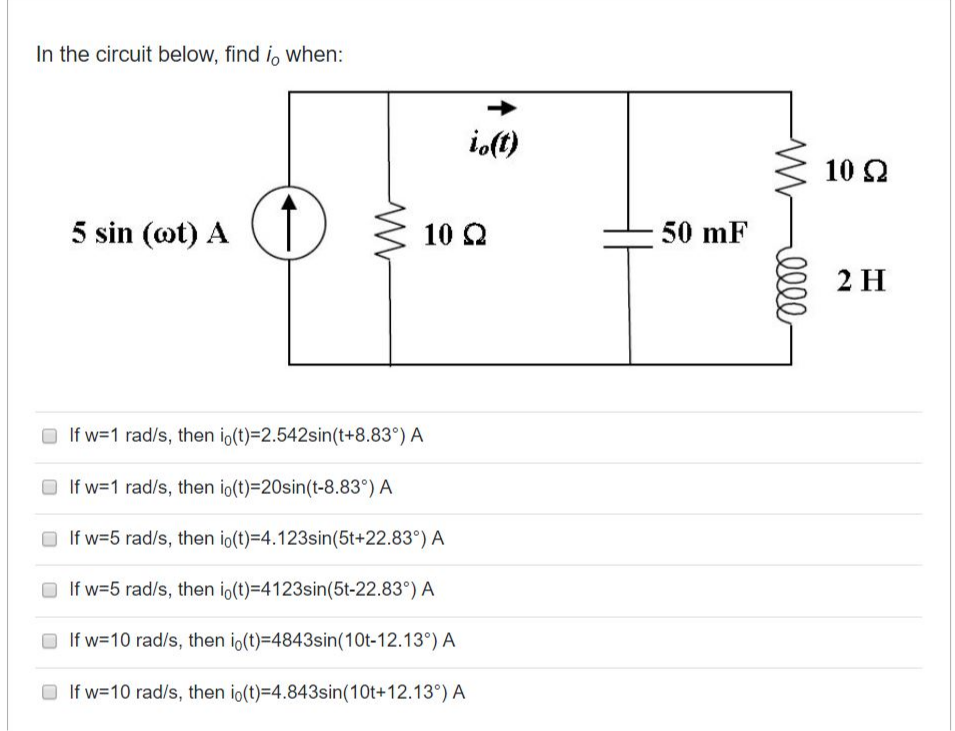# In the circuit below, find i, when: io(t) 10 2 5 sin (ot) A 10 2 50 mF 2 H O If w=1 rad/s, then io(t)=2.542sin(t+8.83°) A O If w=1 rad/s, then io(t)=20sin(t-8.83°) A If w=5 rad/s, then io(t)=4.123sin(5t+22.83°) A O If w=5 rad/s, then io(t)=4123sin(5t-22.83°) A O If w=10 rad/s, then io(t)=4843sin(10t-12.13°) A If w=10 rad/s, then io(t)=4.843sin(10t+12.13°) A

Questionhelp_outlineImage TranscriptioncloseIn the circuit below, find i, when: io(t) 10 2 5 sin (ot) A 10 2 50 mF 2 H O If w=1 rad/s, then io(t)=2.542sin(t+8.83°) A O If w=1 rad/s, then io(t)=20sin(t-8.83°) A If w=5 rad/s, then io(t)=4.123sin(5t+22.83°) A O If w=5 rad/s, then io(t)=4123sin(5t-22.83°) A O If w=10 rad/s, then io(t)=4843sin(10t-12.13°) A If w=10 rad/s, then io(t)=4.843sin(10t+12.13°) A fullscreen

### Want to see this answer and more?

Experts are waiting 24/7 to provide step-by-step solutions in as fast as 30 minutes!*

*Response times vary by subject and question complexity. Median response time is 34 minutes and may be longer for new subjects.
Tagged in
EngineeringElectrical Engineering

### Circuits